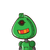# Find the values of x and y in eacha.40N50°70°​

Find the values of x and y in each
a.
40
N
50°
70°​

### 1 thought on “Find the values of x and y in each<br />a.<br />40<br />N<br />50°<br />70°​”

1.By using external angle property

x+50

o

=120

o

∴x=70

o

Sum of all angles of a Δle is 180

o

50

o

+x+y=180

o

50

o

+70

o

+y=180

o

∴y=60

o